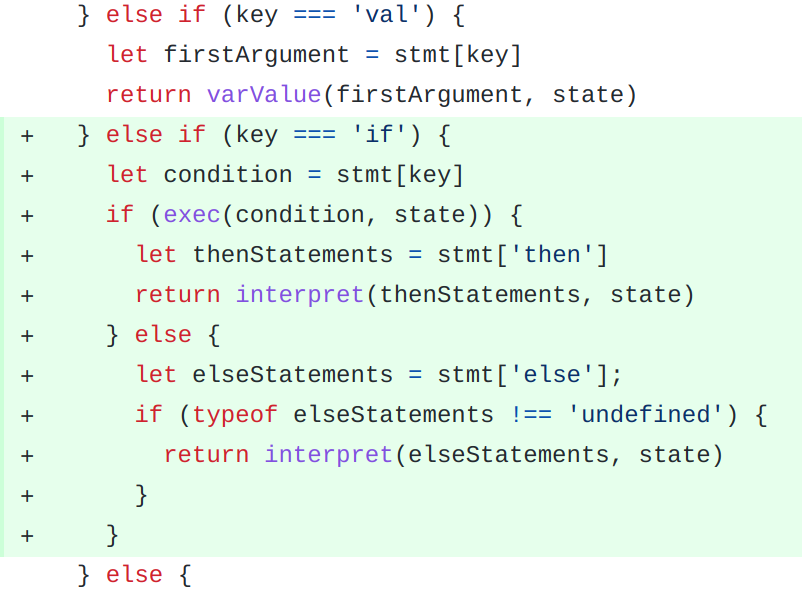# Return Zero

## LISPy-JSON 5: Implement If-Else in an interpreter

Mr Silva

This post is a continuation of LISPy-JSON Part 1Part 2Part-3 and Part-4 you should read these prior to reading the current post.

If-else is nothing but an extension of functions that implement binary operators. With a binary operator you evaluate the two expressions and operate on the result.

With the If-Else statement, you have three expressions:

1. The Condition Expression
2. Then Expression(s)
3. Else Expression(s)

If the result of the condition expression is true, then you evaluate the Then Expression, else you evaluate the Else Expression,If you notice above, our if-else statement is able to return values. This isn’t possible with Javascript but LISPy-JSON supports it. This will allow us to have really short constructs like the following:
``````result = if(condition){
/* result of then */
} else {
/* result of else */
}``````

To make this happen, we will also have to modify the original interpret function to return values

The complete diff can be seen here: https://github.com/avierr/LISPy-JSON-interpreter/commit/d285a22c729958aeaba3283746d519571b7567b9

Just to clean things up, we will refactor out the if-else implementation into it’s own function,

The diff for this can be here: https://github.com/avierr/LISPy-JSON-interpreter/commit/427ff1b9e276da2c69b1824c1e3de7e44006f07c

The entire code for the program is given below:

```let program = [
{ def: { 'a': 10 } },
{ def: { 'b': 20 } },
{
if: { '>': [{ val: 'a' }, { val: 'b' }] },
then: [{ print: 'a is greater than b' }],
else: [{ print: 'b is greater than a' }]
}
]

let builtins = {
print: (text) => { console.log(text) }
}

let binaryOperators = {
'+': (a, b) => { return a + b },
'-': (a, b) => { return a - b },
'*': (a, b) => { return a * b },
'>': (a, b) => { return a > b },
'<': (a, b) => { return a < b },
'==': (a, b) => { return a == b },
'||': (a, b) => { return a || b },
'&&': (a, b) => { return a && b },
}

interpret = (program, state) => {
let returnValue;
for (let stmt of program) {
returnValue = exec(stmt, state)
}
return returnValue;
};

varDefinition = (defObj, state) => {
let variable = Object.keys(defObj);
state[variable] = exec(defObj[variable], state)
}

varValue = (varKey, state) => { return state[varKey] }

let ifStatement = (stmt, key, state) => {
let condition = stmt[key]
if (exec(condition, state)) {
let thenStatements = stmt['then']
return interpret(thenStatements, state)
} else {
let elseStatements = stmt['else'];
if (typeof elseStatements !== 'undefined') {
return interpret(elseStatements, state)
}
}
}

exec = (stmt, state) => {

if (typeof stmt === 'number' || typeof stmt === 'string') {
return stmt;
}

let key = Object.keys(stmt)
if (typeof builtins[key] === 'function') {
builtins[key](exec(stmt[key], state), state)
} else if (typeof binaryOperators[key] === 'function') {
let firstArgument = stmt[key];
let secondArgument = stmt[key];
return binaryOperators[key](exec(firstArgument, state), exec(secondArgument, state))
} else if (key === 'def') {
let firstArgument = stmt[key]
return varDefinition(firstArgument, state)
} else if (key === 'val') {
let firstArgument = stmt[key]
return varValue(firstArgument, state)
} else if (key === 'if') {
return ifStatement(stmt, key, state)
} else {
console.error('unknown instruction: ' + key)
}
}

interpret(program, {})```

The program output is shown below:

``````\$ node interpret.js
b is greater than a``````

The next post will focus on how to implement simple functions in our interpreter.

Tags:
Subscribe
Notify of